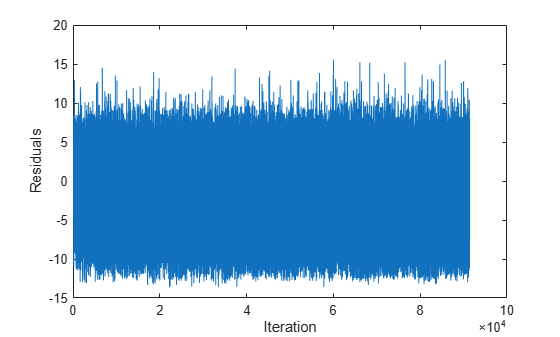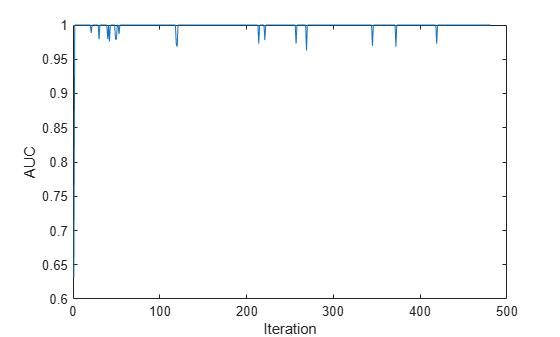predict

Predict responses for new observations from linear model for incremental learning

Description

example

label = predict(Mdl,X) returns the predicted responses or labels label of the observations in the predictor data X from the incremental learning model Mdl.

example

label = predict(Mdl,X,'ObservationsIn',dimension) specifies the observation dimension of the predictor data, either 'rows' (default) or 'columns'. For example, specify 'ObservationsIn','columns' to indicate that observations in the predictor data are oriented along the columns of X.

example

[label,score] = predict(___) also returns classification scores for all classes when Mdl is an incremental learning model for classification, using any of the input argument combinations in the previous syntaxes.

Examples

collapse all

Load the human activity data set.

For details on the data set, enter Description at the command line.

Responses can be one of five classes: Sitting, Standing, Walking, Running, or Dancing. Dichotomize the response by identifying whether the subject is moving (actid > 2).

Y = actid > 2;

Fit a linear classification model to the entire data set.

TTMdl = fitclinear(feat,Y)
TTMdl =
ClassificationLinear
ResponseName: 'Y'
ClassNames: [0 1]
ScoreTransform: 'none'
Beta: [60×1 double]
Bias: -0.2005
Lambda: 4.1537e-05
Learner: 'svm'

Properties, Methods

TTMdl is a ClassificationLinear model object representing a traditionally trained linear classification model.

Convert the traditionally trained linear classification model to a binary classification linear model for incremental learning.

IncrementalMdl = incrementalLearner(TTMdl)
IncrementalMdl =
incrementalClassificationLinear

IsWarm: 1
Metrics: [1×2 table]
ClassNames: [0 1]
ScoreTransform: 'none'
Beta: [60×1 double]
Bias: -0.2005
Learner: 'svm'

Properties, Methods

IncrementalMdl is an incrementalClassificationLinear model object prepared for incremental learning using SVM.

• The incrementalLearner function initializes the incremental learner by passing learned coefficients to it, along with other information TTMdl learned from the training data.

• IncrementalMdl is warm (IsWarm is 1), which means that incremental learning functions can start tracking performance metrics.

• The incrementalLearner configures the model to be trained using the adaptive scale-invariant solver, whereas fitclinear trained TTMdl using the BFGS solver

An incremental learner created from converting a traditionally trained model can generate predictions without further processing.

Predict class labels for all observations using both models.

ttlabels = predict(TTMdl,feat);
illables = predict(IncrementalMdl,feat);
sameLabels = sum(ttlabels ~= illables) == 0
sameLabels = logical
1

Both models predict the same labels for each observation.

If you orient the observations along the columns of the predictor data matrix, you can experience an efficiency boost during incremental learning.

Load and shuffle the 2015 NYC housing data set. For more details on the data, see NYC Open Data.

rng(1) % For reproducibility
n = size(NYCHousing2015,1);
shuffidx = randsample(n,n);
NYCHousing2015 = NYCHousing2015(shuffidx,:);

Extract the response variable SALEPRICE from the table. Apply the log transform to SALEPRICE.

Y = log(NYCHousing2015.SALEPRICE + 1); % Add 1 to avoid log of 0
NYCHousing2015.SALEPRICE = [];

Create dummy variable matrices from the categorical predictors.

catvars = ["BOROUGH" "BUILDINGCLASSCATEGORY" "NEIGHBORHOOD"];
dumvarstbl = varfun(@(x)dummyvar(categorical(x)),NYCHousing2015,...
'InputVariables',catvars);
dumvarmat = table2array(dumvarstbl);
NYCHousing2015(:,catvars) = [];

Treat all other numeric variables in the table as linear predictors of sales price. Concatenate the matrix of dummy variables to the rest of the predictor data, and transpose the data to speed up computations.

idxnum = varfun(@isnumeric,NYCHousing2015,'OutputFormat','uniform');
X = [dumvarmat NYCHousing2015{:,idxnum}]';

Configure a linear regression model for incremental learning with no estimation period.

Mdl = incrementalRegressionLinear('Learner','leastsquares','EstimationPeriod',0);

Mdl is an incrementalRegressionLinear model object.

Perform incremental learning and prediction by following this procedure for each iteration:

• Simulate a data stream by processing a chunk of 100 observations at a time.

• Fit the model to the incoming chunk of data. Specify that the observations are oriented along the columns of the data. Overwrite the previous incremental model with the new model.

• Predict responses using the fitted model and the incoming chunk of data. Specify that the observations are oriented along the columns of the data.

% Preallocation
numObsPerChunk = 100;
n = numel(Y);
nchunk = floor(n/numObsPerChunk);
r = nan(n,1);

figure
h = plot(r);
h.YDataSource = 'r';
ylabel('Residuals')
xlabel('Iteration')

% Incremental fitting
for j = 2:nchunk
ibegin = min(n,numObsPerChunk*(j-1) + 1);
iend   = min(n,numObsPerChunk*j);
idx = ibegin:iend;
Mdl = fit(Mdl,X(:,idx),Y(idx),'ObservationsIn','columns');
yhat = predict(Mdl,X(:,idx),'ObservationsIn','columns');
r(idx) = Y(idx) - yhat;
refreshdata
drawnow
endMdl is an incrementalRegressionLinear model object trained on all the data in the stream.

The residuals appear symmetrically spread around 0 throughout incremental learning.

To compute posterior class probabilities, specify a logistic regression incremental learner.

Load the human activity data set. Randomly shuffle the data.

n = numel(actid);
rng(10); % For reproducibility
idx = randsample(n,n);
X = feat(idx,:);
Y = actid(idx);

For details on the data set, enter Description at the command line.

Responses can be one of five classes: Sitting, Standing, Walking, Running, or Dancing. Dichotomize the response by identifying whether the subject is moving (actid > 2).

Y = Y > 2;

Create an incremental logistic regression model for binary classification. Prepare it for predict by specifying the class names and arbitrary coefficient and bias values.

p = size(X,2);
Beta = randn(p,1);
Bias = randn(1);
Mdl = incrementalClassificationLinear('Learner','logistic','Beta',Beta,...
'Bias',Bias,'ClassNames',unique(Y));

Mdl is an incrementalClassificationLinear model. All its properties are read-only. Instead of specifying arbitrary values, you can take either of these actions to prepare the model:

• Train a logistic regression model for binary classification using fitclinear on a subset of the data (if available), and then convert the model to an incremental learner by using incrementalLearner.

• Incrementally fit Mdl to data by using fit.

Simulate a data stream, and perform the following actions on each incoming chunk of 50 observations.

1. Call predict to predict classification scores for the observations in the incoming chunk of data. The classification scores are posterior class probabilities for logistic regression learners.

2. Call perfcurve to compute the area under the ROC curve (AUC) using the incoming chunk of data, and store the result.

3. Call fit to fit the model to the incoming chunk. Overwrite the previous incremental model with a new one fitted to the incoming observation.

numObsPerChunk = 50;
nchunk = floor(n/numObsPerChunk);
auc = zeros(nchunk,1);

% Incremental learning
for j = 1:nchunk
ibegin = min(n,numObsPerChunk*(j-1) + 1);
iend   = min(n,numObsPerChunk*j);
idx = ibegin:iend;
[~,posteriorProb] = predict(Mdl,X(idx,:));
[~,~,~,auc(j)] = perfcurve(Y(idx),posteriorProb(:,2),Mdl.ClassNames(2));
Mdl = fit(Mdl,X(idx,:),Y(idx));
end

Mdl is an incrementalClassificationLinear model object trained on all the data in the stream.

Plot the AUC on the incoming chunks of data.

plot(auc)
ylabel('AUC')
xlabel('Iteration')The AUC suggests that the classifier correctly predicts moving subjects well.

Input Arguments

collapse all

Incremental learning model, specified as an incrementalClassificationLinear or incrementalRegressionLinear model object. You can create Mdl directly or by converting a supported, traditionally trained machine learning model using the incrementalLearner function. For more details, see the corresponding reference page.

You must configure Mdl to predict labels for a batch of observations.

• If Mdl is a converted, traditionally trained model, you can predict labels without any modifications.

• Otherwise, Mdl must satisfy the following criteria, which you can specify directly or by fitting Mdl to data using fit or updateMetricsAndFit.

• If Mdl is an incrementalRegressionLinear model, its model coefficients Mdl.Beta and bias Mdl.Bias must be nonempty arrays.

• If Mdl is an incrementalClassificationLinear model, its model coefficients Mdl.Beta and bias Mdl.Bias must be nonempty arrays and the class names in Mdl.ClassNames must contain two classes.

• Regardless of object type, if you configure the model so that functions standardize predictor data, the predictor means Mdl.Mu and standard deviations Mdl.Sigma must be nonempty arrays.

Batch of predictor data for which to predict labels, specified as a floating-point matrix of n observations and Mdl.NumPredictors predictor variables. The value of dimension determines the orientation of the variables and observations.

The length of the observation labels Y and the number of observations in X must be equal; Y(j) is the label of observation j (row or column) in X.

Note

predict supports only floating-point input predictor data. If the input model Mdl represents a converted, traditionally trained model fit to categorical data, use dummyvar to convert each categorical variable to a numeric matrix of dummy variables, and concatenate all dummy variable matrices and any other numeric predictors. For more details, see Dummy Variables.

Data Types: single | double

Predictor data observation dimension, specified as 'columns' or 'rows'.

Example: 'ObservationsIn','columns'

Data Types: char | string

Output Arguments

collapse all

Predicted responses (or labels), returned as a categorical or character array; floating-point, logical, or string vector; or cell array of character vectors with n rows. n is the number of observations in X, and label(j) is the predicted response for observation j.

• For classification problems, label has the same data type as the class names stored in Mdl.ClassNames. (The software treats string arrays as cell arrays of character vectors.)

• For regression problems, label is a floating-point vector.

Classification scores, returned as an n-by-2 floating-point matrix when Mdl is an incrementalClassificationLinear model. n is the number of observations in X. score(j,k) is the score for classifying observation j into class k. Mdl.ClassNames specifies the order of the classes.

If Mdl.Learner is 'svm', predict returns raw classification scores. If Mdl.Learner is 'logistic', classification scores are posterior probabilities.

collapse all

Classification Score

For linear incremental learning models for binary classification, the raw classification score for classifying the observation x, a row vector, into the positive class is

$f\left(x\right)={\beta }_{0}+x\beta ,$

where

• β0 is the scalar bias Mdl.Bias.

• β is the column vector of coefficients Mdl.Beta.

The raw classification score for classifying x into the negative class is –f(x). The software classifies observations into the class that yields the positive score.

If the linear classification model consists of logistic regression learners, then the software applies the 'logit' score transformation to the raw classification scores.